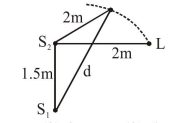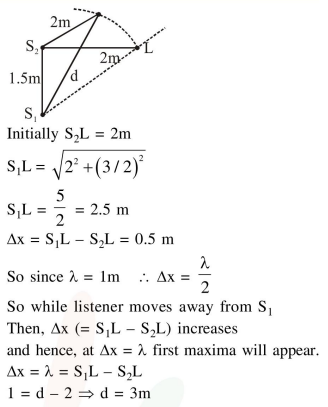# Two coherent sources of sound,`
Question:

Two coherent sources of sound, $S_{1}$ and $S_{2}$, produce sound waves of the same wavelength, $\lambda=1 \mathrm{~m}$, in phase.

$\mathrm{S}_{1}$ and $\mathrm{S}_{2}$ are placed $1.5 \mathrm{~m}$ apart (see fig.) A listener, located at $\mathrm{L}$, directly in front of $S_{2}$ fi

nds that the intensity is at a minimum when he is $2 \mathrm{~m}$ away from $\mathrm{S}_{2}$. The listener moves away from $S_{1}$, keeping his

distance from $S_{2}$ fixed. The adjacent maximum of intensity is observed when the listener is at a distance $d$ from $S_{1}$. Then, $d$ is :1. $12 \mathrm{~m}$

2. $3 \mathrm{~m}$

3. $5 \mathrm{~m}$

4. $2 \mathrm{~m}$

Correct Option: , 2

Solution: